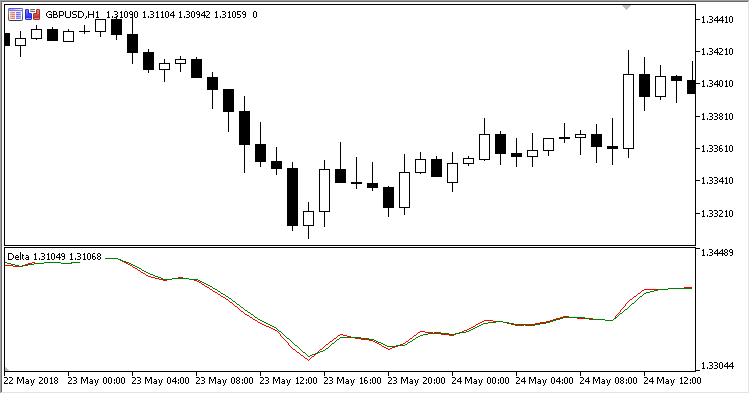Interesting script?
So post a link to it -
let others appraise it

You liked the script? Try it in the MetaTrader 5 terminal# Delta - indicator for MetaTrader 5

Views:
1288
Rating:
Published:
2018.09.27 15:27

The Delta indicator draws two lines:

• Red - the bar average price line (OHLC)/4;
• Green - the line of the decimal logarithm of the current to previous (OHLC)/4 prices ratio.

The indicator has no input parameters.

Calculations:

`Delta = Price + Diff`

where:

```Diff = Log10(PrevPrice / Price)
Price = (Open + High + Low + Close) / 4.0
```Translated from Russian by MetaQuotes Software Corp.
Original code: https://www.mql5.com/ru/code/21711Darvas_Box

A channel filter indicator Darvas Box.Damiani_Volatmeter

Damiani Volatmeter - trend/flat determining algorithm.Delta_Oscillator

The Delta Oscillator indicator displays the difference in the Delta indicator lines as a histogram.Deviation_Oscillator

Deviation Oscillator - a volatility oscillator which represents a difference between the price and the MA, normalized within the selected range.